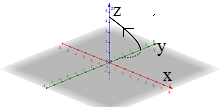# Neatly sketch the curve with the vector equation r^→(t) = (-t^2, 4, t) . Identify any...

## Question:

Neatly sketch the curve with the vector equation {eq}r^→(t) = (-t^2, 4, t) {/eq}. Identify any special points on its graph and indicate with an arrow the orientation of this curve.

## Parametric curve in space:

A parametric curve in space is described by the vector function

{eq}\vec r(t) = (x(t),y(t),z(t)) {/eq}.

where t is the parameter.

The sketch of the curve in space can be produced by plotting the sequence of points (x,y,z) as t varies.

The sketch of the parametric curve with the vector equation {eq}\vec r(t) = (-t^2, 4, t) {/eq}

is obtained using computer technology and reported in the figure below with the

arrow indicating the direction where t increases.

The curve is a parabola whose vertex is the point (0,4,0).